#### Generalized Coefficient of Determination

Cox and Snell (1989, pp. 208–209) propose the following generalization of the coefficient of determination to a more general linear model: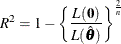where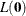is the likelihood of the intercept-only model,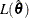is the likelihood of the specified model, and n is the sample size. The quantity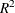achieves a maximum of less than 1 for discrete models, where the maximum is given by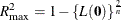Nagelkerke (1991) proposes the following adjusted coefficient, which can achieve a maximum value of 1: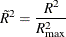Properties and interpretation ofand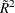are provided in Nagelkerke (1991). In the Testing Global Null Hypothesis: BETA=0 table,is labeled as RSquare andis labeled as Max-rescaled RSquare.  Use the RSQUARE option to requestand.